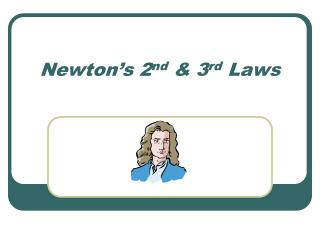DownloadDownload PresentationNewton’s 2 nd & 3 rd Laws

# Newton’s 2 nd & 3 rd Laws

Download Presentation## Newton’s 2 nd & 3 rd Laws

- - - - - - - - - - - - - - - - - - - - - - - - - - - E N D - - - - - - - - - - - - - - - - - - - - - - - - - - -
##### Presentation Transcript

1. Newton’s 2nd & 3rd Laws

2. A couple things to keep straight • Mass = the amount of “stuff” something is made of. • Weight = the measure of the force of gravity on an object.

3. A couple of things to remember • You can use Newton’s 2nd Law to solve for weight. • Weight = force of gravity acting on a specific object. (acceleration due to gravity is -9.8m/s2 ) therefore…

4. How to solve for weight • Fnet = m x a (2nd Law) • Weight = mass of object x acc. due to gravity • F(grav) = m x acc (due to “g”) • Weight = m x acc (due to “g”) so: F(grav) = weight (Good formula to remember = F(grav) = m x g)

5. Standard Units of Force • Force measured in Newtons (N) • 1 N = 1 kg x m/s2 • 1 Kg weighs approximately 2.2 lbs • 1 kg weighs approximately 10 N • So…10 N = 2.2 lbs

6. Solving a Force Problem with Newton’s 2nd Law • Fnet= sum of all forces • If there is no motion in one direction (“X” or “Y”), forces are balanced…nothing to solve. • Must determine direction of motion and solve for those net forces • Fnet= Fapp - Ff OR Fnet = Fn – Fg

7. A question to make you think??? • Is the force of gravity the same for all objects on Earth? Answer… NO!!! Due to mass, however acceleration is the same!

8. A couple things about Newton’s 3rd Law • Forces always occur in pairs • “For every action there is an equal & opposite reaction” Fa on b = -Fb on a Ex. Car driving down the road. What are the forces?

9. A couple things about Newton’s 3rd Law (cont.) What forces are acting on the car? Force of grav. (down) / Normal Force (up) Force of friction (road on car) / Force of friction (car on road)

10. A couple things about Newton’s 3rd Law (cont.) • Drop a softball (mass 0.18Kg) ? What is the force on Earth due to the ball ? What is the Earth’s acceleration (mass of Earth = 6.0 x 1024 Kg) hint: use Fg = mg

11. Softball Answer • F(grav) = (0.18 kg) x (-9.8 m/s2) F = -1.8 N (therefore, force on ball from Earth = 1.8 • Acceleration = 1.8 N = (6.0E24)a 3.1E-25 m/s2